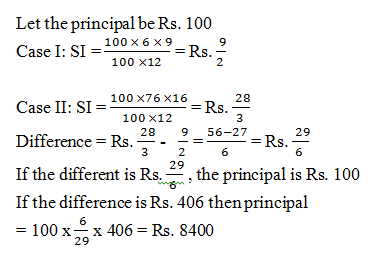# Mixed Quantitative Aptitude Test for IBPS PO Mains - Part 5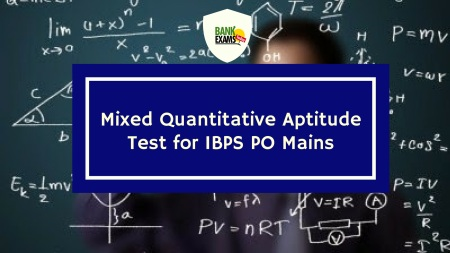#### Ques 1.

P and Q can complete a job in 24 days working together. P alone can complete it in 32 days. Both of them worked together for 8 days and then P left. The number of days Q will talk to complete the remaining work is
1. 62
2. 60
3. 65
4. 64
5. 66

#### Ques 2.

A passenger sitting in a train of a certain length which is running at a speed of 60 km/hr passing through two bridges. the notices that be crossed the first bridge and second bridge in time intervals which are in the ratio of 7:4 respectively. If the length of the first bridge is 350m, then the length of the second bridge is
1. 210m
2. 220m
3. 200 m
4. 190m
5. 180m

#### Ques 3.

The average salary per head of all workers of an institution is Rs. 80. The average salary per head of 16 officers is Rs. 600. The average salary per head of 16 officers is Rs. 600. The average salary per head of the rest is Rs. 67. Then, the total number of workers in the institution is
1. 656
2. 665
3. 655
4. 676
5. 666

#### Ques 4.

Four horses are tethered at 4 corners of a square field so that they just reach one another. If the side of the square field is 56m, what will be the area left ungrazed?
1. 670m²
2. 627m²
3. 634m²
4. 684m²
5. 672m²

#### Ques 5.

In a town, each of the 60% of families has a laptop, each of the 30% of families has a computer and each of the 15% of families has both a laptop and a computer. In all, there are 10800 families in the town. How many families in the town do not have a laptop or a computer?
1. 2600
2. 2850
3. 2900
4. 2650
5. 2700

#### Ques 6.

An article of cost prince Rs. 16000 is marked at Rs. 19200. After allowing a discount of x% a profit of 15% is made. The value of x is.
1. 3.17%
2. 4.17%
3. 5.17%
4. 2.75%
5. 6.25%

#### Ques 7.

A box contains 4 red, 5 black and 6 green marbles. Three marbles are drawn at random, what is the probability that all the marbles drawn are of the same colour?
1. 17/455
2. 438/455
3. 34/455
4. 421/455
5. Other than the given options

#### Ques 8.

P,Q and R started a business by investing Rs. 40,500, Rs. 45000 and Rs. 50000 respectively. After 6 months R withdrew Rs. 10,000 while P invested Rs. 4500 more. In annual profit of Rs. 53,100 the share of R will exceed that of P by
1. Rs. 1000
2. Rs. 850
3. Rs. 950
4. Rs. 800
5. Rs. 900

#### Ques 9.

Three taps X,Y and Z can fill a tank in 12 hrs, 15 hrs and 20 hrs respectively. If X is open all the time and Y and Z are open for one hour each alternately, starting with Y, then the tank will be full in how many hours?
1. 10 hr
2. 6 hr
3. 8 hr
4. 7 hr
5. 9 hr

#### Ques 10.

If the simple interest on a certain sum of money for 9 months at 6% is Rs. 406 less than the simple interest on the same sum for 16 months at 7% pa, then find the sum.
1. Rs. 8600
2. Rs. 8400
3. Rs. 8800
4. Rs. 8900
5. Rs. 9000

1. 4;2. 3;
The length of the train in which passenger is sitting is not considered since we are concerned with the passenger instead of train.
∴ Let the length of the second bridge be x m3. 1;4. 5;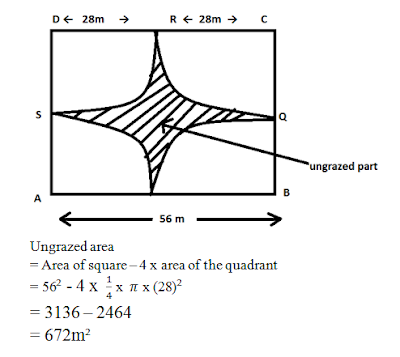5. 5;
The percentage of families having either a laptop or a computer or both
= 60+30 -15 = 75%
Families having neither a laptop nor a computer
= 100 – 75 = 25%
So, required family = 25/100 x 10800 = 2700

6. 2;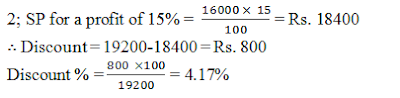7. 3;8. 5;

 P Q R First 6 months 40500 x 6 45000 x 6 50000 x 6 Last 6 months 45000 x 6 45000 x 6 40000 x 6 513000 540000 540000

Ratio of their profits = 513 : 540 : 540
= 57:60:60 = 19:20:20
Share of R will exceed that of P by9. 4;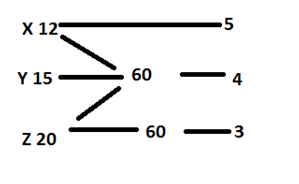Work is progressing (X+Y) + (X+Z) + (X+Y) + (X+Z)+ ……..
Work done in two hours = (5+4) + (5+3) = 17
∴ Work done in 6 hrs = 17x 6/2 = 51 units
∴ Work done in 7 hours = 51+9=60 units
So, work will be completed in 7 hours.

10. 2;